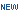# 数据表

#### 数据表 用于  Digital Transistors (BRTs) (显示所有)

1 - 50 of 146    [  1  2 3  下一页 ]
 Document TitleBias Resistor Transistor DTA144TT1/D (124.0kB) 3
Complementary Bias Resistor TransistorsDTC143JP/D (99kB) 2 Aug, 2021
Complementary Bias Resistor Transistors MUN531335DW1/D (75kB) 2 Nov, 2016
Complementary Bias Resistor Transistors R1 = 1 kΩ, R2 = 1 kΩ DTC113EP/D (77kB) 3 Jul, 2014
Complementary Bias Resistor Transistors R1 = 10 kΩ, R2 = ∞ kΩ DTC114TP/D (111.0kB) 0 Sep, 2012
Complementary Bias Resistor Transistors R1 = 10 kΩ, R2 = 10 kΩ DTC114EP/D (98kB) 4 Jul, 2018
Complementary Bias Resistor Transistors R1 = 10 kΩ, R2 = 47 kΩ DTC114YP/D (135kB) 6 Jun, 2017
Complementary Bias Resistor Transistors R1 = 100 kOhm, R2 = 100 kOhm DTC115EP/D (78kB) 1 Oct, 2017
Complementary Bias Resistor Transistors R1 = 100 kW, R2 = 8 kW DTC115TP/D (129kB) 1 Jun, 2017
Complementary Bias Resistor Transistors R1 = 2.2 kΩ, R2 = ∞ kΩ DTC123TP/D (129kB) 1 Jun, 2017
Complementary Bias Resistor Transistors R1 = 2.2 kΩ, R2 = 2.2 kΩ DTC123EP/D (74kB) 1 Nov, 2014
Complementary Bias Resistor Transistors R1 = 2.2 kΩ, R2 = 47 kΩ DTC123JP/D (135kB) 4 Jun, 2017
Complementary Bias Resistor Transistors R1 = 22 kΩ, R2 = 22 kΩ DTC124EP/D (99kB) 5 Oct, 2017
Complementary Bias Resistor Transistors R1 = 22 kOhm, R2 = 47 kOhm DTC124XP/D (145kB) 2 Mar, 2016
Complementary Bias Resistor Transistors R1 = 4.7 kΩ, R2 = 4.7 kΩ DTC143EP/D (164kB) 2 Jun, 2017
Complementary Bias Resistor Transistors R1 = 4.7 kΩ, R2 = 47 kΩ DTC143ZP/D (142kB) 7 Apr, 2021
Complementary Bias Resistor Transistors R1 = 4.7 kΩ, R2 = 10 kΩMUN5338/D (118kB) 1 Jul, 2021
Complementary Bias Resistor Transistors R1 = 4.7 kOhm, R2 = inf kOhm DTC143TP/D (77kB) 3 Mar, 2016
Complementary Bias Resistor Transistors R1 = 47 kΩ, R2 = 22 kΩ DTC144WP/D (125kB) 1 Jun, 2017
Complementary Bias Resistor Transistors R1 = 47 kΩ, R2 = 47 kΩ DTC144EP/D (100kB) 4 Jun, 2017
Digital Bias Resistor Transistor MMUN2218L/D (117kB) 1 Oct, 2016
Digital TransistorsDTC114E/D (102kB) 9 Aug, 2021
Digital Transistors (BRT) DTA123J/D (103kB) 5 Apr, 2017
Digital Transistors (BRT) DTA144W/D (178kB) 5 Oct, 2016
Digital Transistors (BRT) DTA124X/D (137kB) 6 Mar, 2019
Digital Transistors (BRT) R1 = 1 kOhm, R2 = 1 kOhm DTC113E/D (128kB) 4 Nov, 2016
Digital Transistors (BRT) R1 = 1 kOhms, R2 = 1 kOhms DTA113E/D (171kB) 6 Oct, 2016
Digital Transistors (BRT) R1 = 10 kOhm, R2 = 47 kOhm DTA114Y/D (178kB) 6 Oct, 2016
Digital Transistors (BRT) R1 = 10 kOhms, R2 = 10 kOhms DTA114E/D (102kB) 9 Jul, 2018
Digital Transistors (BRT) R1 = 10 kOhms, R2 = 47 kOhms DTC114Y/D (135kB) 4 Nov, 2016
Digital Transistors (BRT) R1 = 10 kOhms, R2 = inf kOhms DTA114T/D (177kB) 3 Oct, 2016
Digital Transistors (BRT) R1 = 100 kOhms, R2 = 100 kOhms DTC115E/D (130kB) 5 Jan, 2019
Digital Transistors (BRT) R1 = 100 kOhms, R2 = 100 kOhms DTA115E/D (88kB) 6 Feb, 2018
Digital Transistors (BRT) R1 = 100 kOhms, R2 = inf kOhms DTC115T/D (128kB) 2 Nov, 2016
Digital Transistors (BRT) R1 = 2.2 kOhm, R2 = 2.2 kOhm DTC123E/D (96kB) 4 Oct, 2016
Digital Transistors (BRT) R1 = 2.2 kOhm, R2 = 47 kOhm, NPN Transistors with Monolithic Bias Resistor Network DTC123J/D (104kB) 5 Mar, 2017
Digital Transistors (BRT) R1 = 2.2 kOhms, R2 = 2.2 kOhms DTA123E/D (170kB) 5 Oct, 2016
Digital Transistors (BRT) R1 = 2.2 kOhms, R2 = inf kOhms DTC123T/D (133kB) 2 Oct, 2016
Digital Transistors (BRT) R1 = 2.2 kOhms, R2 = inf kOhms DTA123T/D (172kB) 4 Oct, 2016
Digital Transistors (BRT) R1 = 22 kOhm, R2 = 22 kOhm DTC124E/D (134kB) 3 Oct, 2016
Digital Transistors (BRT) R1 = 22 kOhm, R2 = 47 kOhm DTC124X/D (128kB) 3 Oct, 2016
Digital Transistors (BRT) R1 = 22 kOhms, R2 = 22 kOhms DTA124E/D (177kB) 6 Oct, 2016
Digital Transistors (BRT) R1 = 4.7 kOHMs, R2 = 10 kOHMs DTC143X/D (57kB) 3 Oct, 2016
Digital Transistors (BRT) R1 = 4.7 kOhms, R2 = 4.7 kOhms DTC143E/D (133kB) 3 Oct, 2016
Digital Transistors (BRT) R1 = 4.7 kOhms, R2 = 4.7 kOhms DTA143E/D (102kB) 6 Nov, 2016
Digital Transistors (BRT) R1 = 4.7 kOhms, R2 = 47 kOhms DTC143Z/D (134kB) 6 Oct, 2016
Digital Transistors (BRT) R1 = 4.7 kOhms, R2 = 47 kOhms DTA143Z/D (177kB) 7 Oct, 2016
Digital Transistors (BRT) R1 = 4.7 kOhms, R2 = inf kOhms DTC143T/D (128kB) 3 Oct, 2016
Digital Transistors (BRT) R1 = 4.7 kOhms, R2 = inf kOhms DTA143T/D (172kB) 3 Oct, 2016
Digital Transistors (BRT) R1 = 47 kOhm, R2 = 22 kOhm DTC144W/D (154kB) 6 Sep, 2019Connect with us